407 493 6601

# Precalculus - Hyperbolas

## Introduction

• Hyperbola is the set of points in a plane wherein the absolute value of the difference of the distances from two fixed points, the foci, is constant. The line joining both points (foci) is called the transverse axis.
• Hyperbola is formed when a cone is intersected by a plane parallel to the x-axis.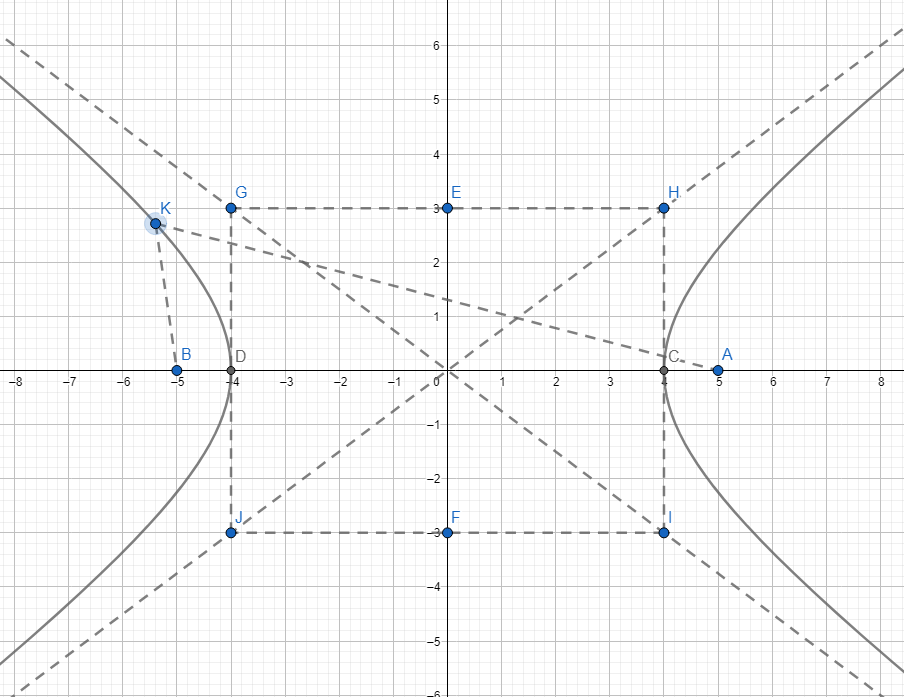The absolute value of the differences of the distances from two fixed points is expressed as $\left|{d}_{1}-{d}_{2}\right|=2a$

In the hyperbola above, we have $\left|AK-BK\right|=2a$

• The general equation of a hyperbola is .
• Its eccentricity is $>1$. The formula to find the eccentricity of the hyperbola is given by $e=\frac{c}{a}$.
• To visualize the properties of a hyperbola, study the graph below: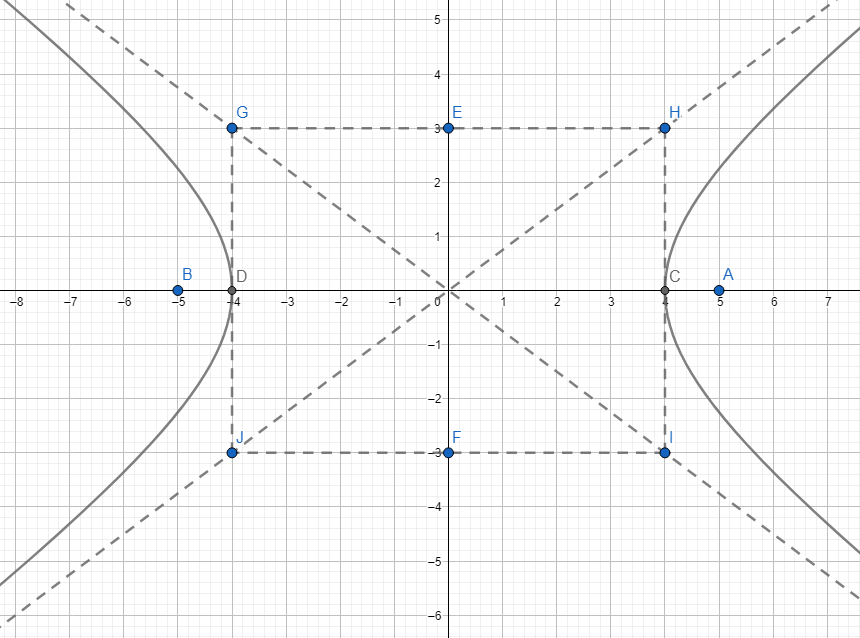• Observe that the hyperbola has an auxiliary rectangle where its two opposite sides intersect the vertices of the curve.
• Two asymptotes are diagonal lines of the auxiliary rectangle whose intersection is the hyperbola's center.
• The curve of the hyperbola is symmetric to the x-axis and y-axis.
• The value of $a$, unlike the ellipse, is not necessarily greater than $b$.
• The hyperbola above has a center at $\left(0,0\right)$, foci at , vertices at , Co-vertices at . The equation of the hyperbola above is $\frac{{x}^{2}}{16}-\frac{{y}^{2}}{9}=1$.
• The asymptotes are $y=±\frac{3}{4}x$.

## Forms and Graphs of Hyperbola

1. Center at $\left(0,0\right)$ with transverse axis along the x-axis (horizontal transverse axis)

Standard Equation is $\frac{{x}^{2}}{{a}^{2}}-\frac{{y}^{2}}{{b}^{2}}=1$

To find the value of c, we have: ${a}^{2}+{b}^{2}={c}^{2}$ (center to focus distance)

The length of the latus rectum is computed using $L=\frac{2{b}^{2}}{a}$.

The asymptotes are $y=±\frac{b}{a}x$

The coordinates of the foci are .

The coordinates of the vertices are .

Coordinates of the co-vertices are

The length of the conjugate axis is $2b$.

Graph: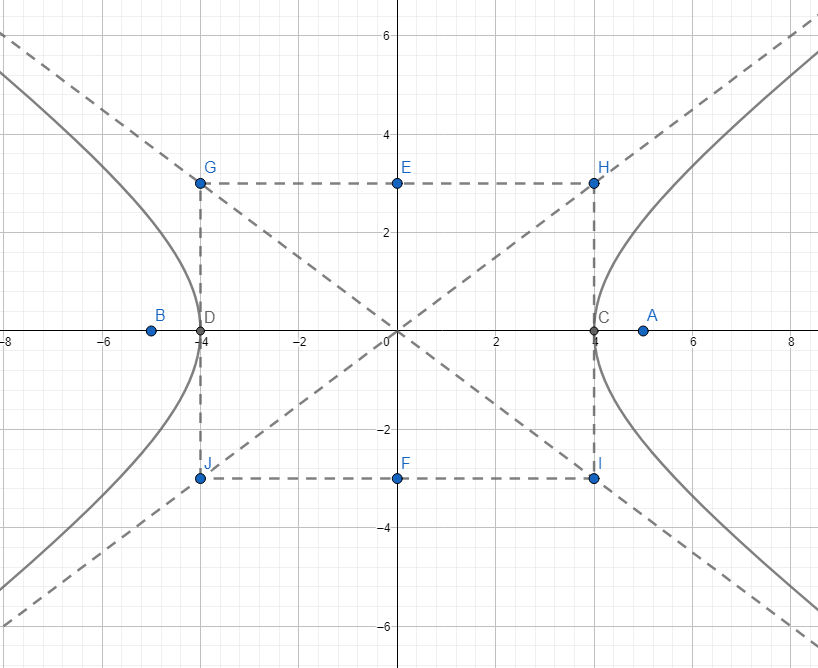2. Center at $\left(0,0\right)$ with a transverse axis along the y-axis (vertical transverse axis)

Standard equation is $\frac{{y}^{2}}{{a}^{2}}-\frac{{x}^{2}}{{b}^{2}}=1$

To find the value of c, we have ${c}^{2}={a}^{2}+{b}^{2}$ (center to focus distance)

The length of the latus rectum is given by $L=\frac{2{b}^{2}}{a}$.

The asymptotes are $y=±\frac{a}{b}x$

The coordinates of the foci are .

Coordinates of the vertices are

Coordinates of the co-vertices are

The length of the conjugate axis is $2b$.

Graph: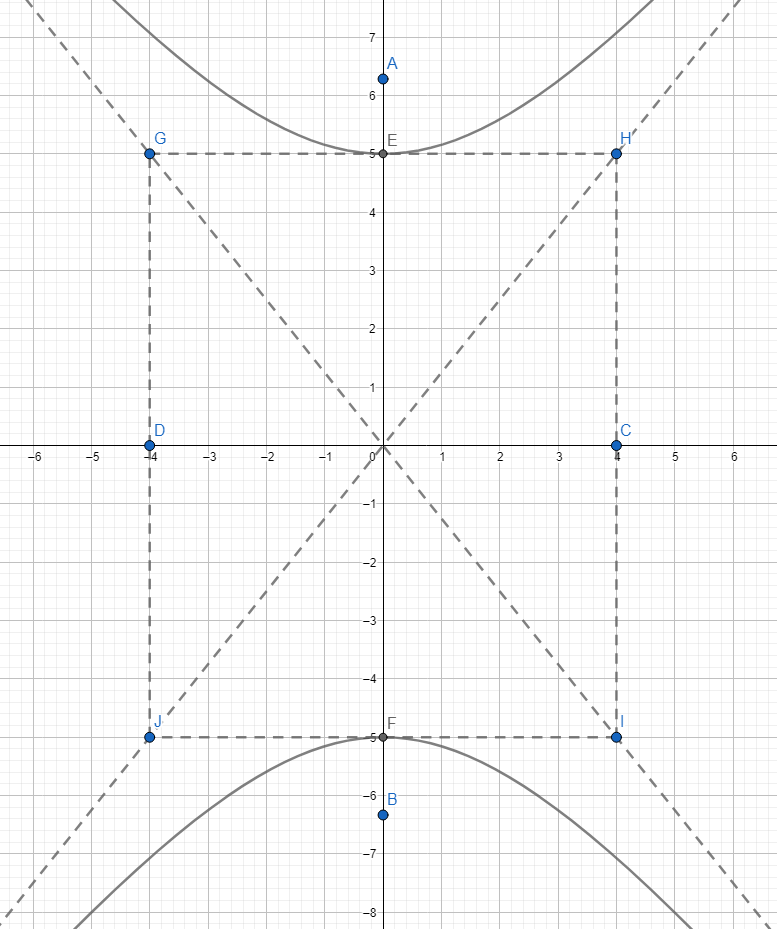3. Center at $\left(h,k\right)$ with a transverse axis parallel to the x-axis

Standard Equation is $\frac{{\left(x-h\right)}^{2}}{{a}^{2}}-\frac{{\left(y-k\right)}^{2}}{{b}^{2}}=1$

Center to focus distance is ${c}^{2}={a}^{2}+{b}^{2}$.

The coordinates of the vertices are .

Coordinates of the foci are

Coordinates of the co-vertices (endpoints of the conjugate axis) are

Asymptotes are $y-k=±\frac{b}{a}\left(x-h\right)$

Graph: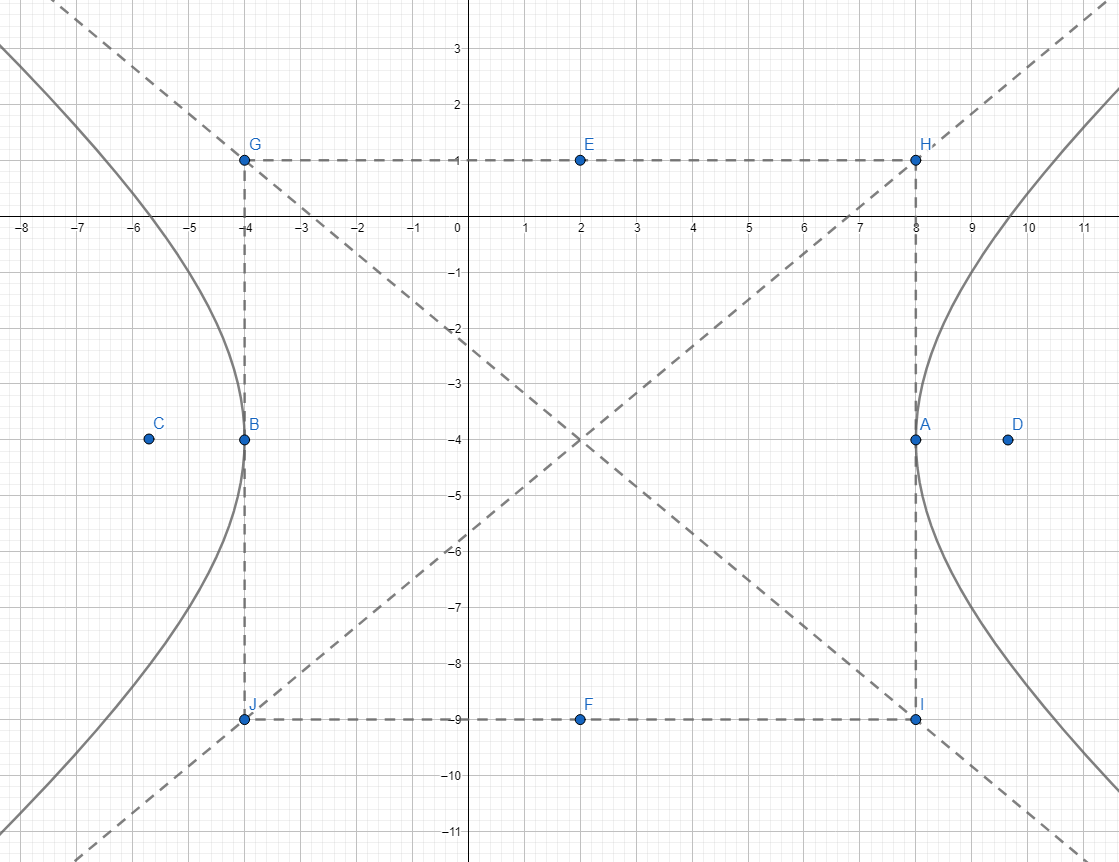4. Center at $\left(h,k\right)$ with a transverse axis parallel to the y-axis.

Standard Equation is $\frac{{\left(y-k\right)}^{2}}{{a}^{2}}-\frac{{\left(x-h\right)}^{2}}{{b}^{2}}=1$

Center to focus distance is ${c}^{2}={a}^{2}+{b}^{2}$

The coordinates of the vertices are .

The coordinates of the foci are .

Coordinates of the co-vertices (endpoints of the conjugate axis) are .

Graph: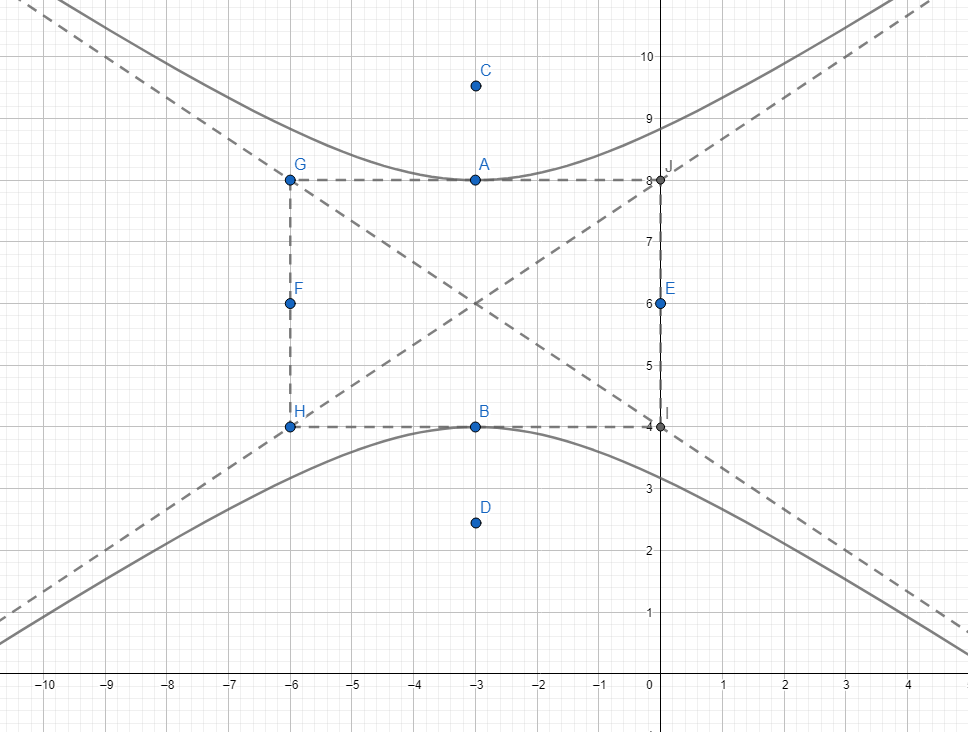## Solved Examples

Example 1. In the hyperbola $\frac{{x}^{2}}{16}-\frac{{y}^{2}}{9}=1$, locate the center, vertices, co-vertices, and foci. What is the equation of the asymptotes?

Solution:

This hyperbola has its transverse axis along the x-axis. The center is at $\left(0,0\right)$.

To solve for $a$, we have ${a}^{2}=16\to a=4$.

To find b, we have ${b}^{2}=9\to b=3$

To find the value of $c$, we use the formula ${c}^{2}={a}^{2}+{b}^{2}$. This gives $c=5$.

Based on the computed values, we have the following coordinates:

Foci at

Vertices at .

Co-vertices at

The equation of the asymptotes is $y=±\frac{b}{a}x\to y=±\frac{3}{4}x$

Example 2. Find the equation of the hyperbola with a horizontal transverse axis, an eccentricity of 4, and a distance between the foci is $4\sqrt{3}$.

Solution:

This hyperbola has a standard equation of $\frac{{x}^{2}}{{a}^{2}}-\frac{{y}^{2}}{{b}^{2}}=1$.

If the distance between the foci is $4\sqrt{3}$, then $2c=4\sqrt{3}\to c=2\sqrt{3}$.

To solve for a, we use eccentricity $e=\frac{c}{a}$.

$4=\frac{2\sqrt{3}}{a}\to 4a=2\sqrt{3}\to a=\frac{\sqrt{3}}{2}$

We use ${c}^{2}={a}^{2}+{b}^{2}$ to solve for $b$. This yields ${b}^{2}=\frac{45}{4}$

Hence, the equation is

Example 3. What is the equation of the hyperbola with vertices at  and one focus of $\left(0,9\right)$?

Solution:

This hyperbola has a transverse axis along the y-axis, which follows the form $\frac{{y}^{2}}{{a}^{2}}-\frac{{x}^{2}}{{b}^{2}}=1$.

If the vertices and one focus are , and $\left(0,9\right)$, then .

Using ${c}^{2}={a}^{2}+{b}^{2}$ yields ${b}^{2}=32$.

Thus, the equation of the hyperbola is $\frac{{y}^{2}}{49}-\frac{{x}^{2}}{32}=1$

Example 4. Determine the center, vertices, co-vertices, and foci of the hyperbola given by $\frac{{\left(x+3\right)}^{2}}{36}-\frac{{\left(y-2\right)}^{2}}{25}=1$.

Solution:

The center is  $\left(-3,2\right)$ where .

Based on the given equation, .

Using the formula ${c}^{2}={a}^{2}+{b}^{2}$, then $c=\sqrt{61}$.

Foci are at

Vertices at

Co-vertices at

Example 5. Find the equation of the hyperbola with center at $\left(-6,5\right)$, foci at , and eccentricity of 6.

Solution:

Based on the given points, the hyperbola has a horizontal transverse axis.

If the foci are at , it follows that  which gives $c=3$.

Using the eccentricity , then $a=\frac{1}{2}$.

Using the formula ${c}^{2}={a}^{2}+{b}^{2}$, it gives ${b}^{2}=\frac{35}{4}$.

Hence, the equation of the hyperbola is

Example 6. Locate the center of the hyperbola ${x}^{2}-4{y}^{2}+4x+32y-96=0$.

Solution:

Use completing the square to determine the coordinates of the center.

${x}^{2}+4x-4{y}^{2}+32y=96$

$\left({x}^{2}+4x+4\right)-4\left({y}^{2}-8y+16\right)=96+4+\left(-4\right)\left(16\right)$

${\left(x+2\right)}^{2}-4{\left(y-4\right)}^{2}=36$, with the center at $\left(-2,4\right)$

## Cheat Sheet

 Standard Forms of Hyperbola Coordinates Equation of the asymptotes Center and transverse axis Vertices Foci Co-vertices (endpoints of the conjugate axis) $\frac{{x}^{2}}{{a}^{2}}-\frac{{y}^{2}}{{b}^{2}}=1$ $\left(0,0\right)$ along the x-axis $\left(±a,0\right)$ $\left(±c,0\right)$ $\left(0,±b\right)$ $y=±\frac{b}{a}x$ $\frac{{y}^{2}}{{a}^{2}}-\frac{{x}^{2}}{{b}^{2}}=1$ $\left(0,0\right)$ along the y-axis $\left(0,±a\right)$ $\left(0,±c\right)$ $y=±\frac{a}{b}x$ $\frac{{\left(x-h\right)}^{2}}{{a}^{2}}-\frac{{\left(y-k\right)}^{2}}{{b}^{2}}=1$ $\left(h,k\right)$ parallel to x-axis $\left(h±a,k\right)$ $\left(h±c,k\right)$ $y-k=±\frac{b}{a}\left(x-h\right)$ $\frac{{\left(y-k\right)}^{2}}{{a}^{2}}-\frac{{\left(x-h\right)}^{2}}{{b}^{2}}=1$ $\left(h,k\right)$ parallel to y-axis $y-k=±\frac{a}{b}\left(x-h\right)$

## Blunder Areas

• In the equation of the hyperbola, $a$ is not necessarily greater than or less than b, unlike in the case of the ellipse.
• Always notice the difference between the hyperbola in terms of the transverse axis and the conjugate axis.
• Be cognizant of the difference between an ellipse and a hyperbola in terms of their standard equation and general equation.